Markov Reward Process

By Prof. Seungchul Lee
http://iai.postech.ac.kr/
Industrial AI Lab at POSTECH

Source

• By David Silver's RL Course at UCL
• By Prof. Zico Kolter at CMU

# 1. Markov Reward Process¶

• Suppose that each transition in a Markov chain is associated with a reward, $r$
• As the Markov chain proceeds from state to state, there is an associated sequence of rewards
• Discount factor $\gamma$
• Later, we will study dynamic programming and Markov decision theory $\implies$ Markov Decision Process (MDP)

## 1.1. Definition of MRP¶

Definition: A Markov Reward Process is a tuple $\langle S,P,R,\gamma \rangle$

• $S$ is a finite set of states
• $P$ is a state transition probability matrix
$$P_{ss'} = P\left[S_{t+1} = s' \mid S_t = s \right]$$
• $R$ is a reward function, $R = \mathbb{E} \left[ R_{t+1} \mid S_t = s \right]$
• $\gamma$ is a discount factor, $\gamma \in [0,1]$

Example: student MRP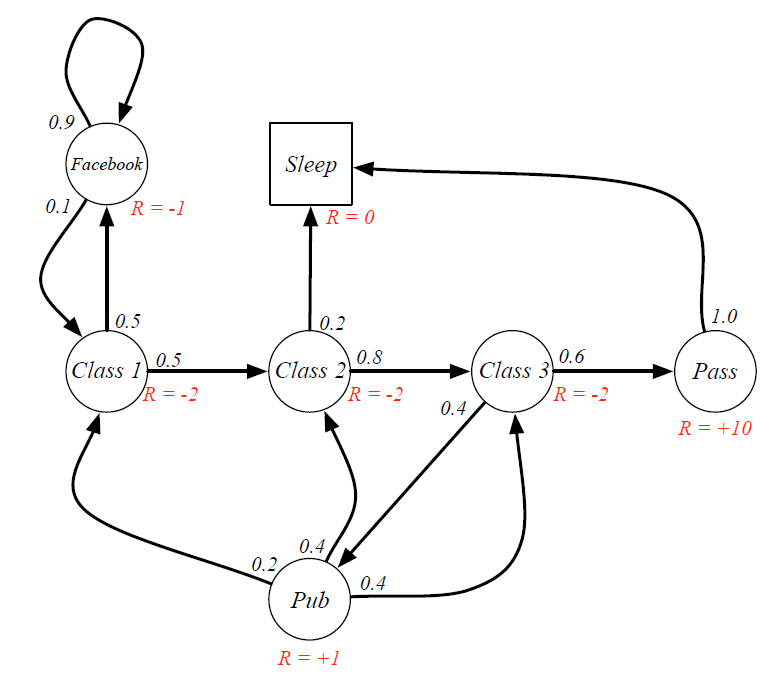## 1.2. Reward over Multiple Transitions (= Return $G_t$)¶

Definition: The return $G_t$ is the total discounted reward from time-step $t$

$$G_t = R_{t+1} + \gamma R_{t+2} + \cdots = \sum_{k=0}^{\infty}\gamma^k R_{t+k+1}$$

Discount factor $\gamma$

• It is reasonable to maximize the sum of rewards
• It is also reasonable to prefer rewards now to rewards later
• One solution: values of rewards decay exponentially
• Mathematically convenient (avoid infinite returns and values)
• Human often acts as if there is a discount factor $\gamma < 1$
• $\gamma = 0$: Only care about immediate reward
• $\gamma = 1$: Future reward is as beneficial as immediate reward
In :
import numpy as np

In :
# [C1 C2 C3 Pass Pub FB Sleep] = [0 1 2 3 4 5 6]

R = [-2, -2, -2, 10, 1, -1, 0]
gamma = 0.9

# if a sequence is given
S = [0, 1, 2, 4, 2, 4]

G = 0

for i in range(6):
G = G + (gamma**i)*R[S[i]]

print(G)

-5.41271

In :
R = [-2, -2, -2, 10, 1, -1, 0]
gamma = 0.9

P = [[0, 0.5, 0, 0, 0, 0.5, 0],
[0, 0, 0.8, 0, 0, 0, 0.2],
[0, 0, 0, 0.6, 0.4, 0, 0],
[0, 0, 0, 0, 0, 0, 1],
[0.2, 0.4, 0.4, 0, 0, 0, 0],
[0.1, 0, 0, 0, 0, 0.9, 0],
[0, 0, 0, 0, 0, 0, 1]]

# sequence generated by Markov chain
# [C1 C2 C3 Pass Pub FB Sleep] = [0 1 2 3 4 5 6]

# starting from 0
x = 0
S = []
S.append(x)

for i in range(5):
x = np.random.choice(len(P),1,p = P[x][:])
S.append(x)

G = 0
for i in range(5):
G = G + (gamma**i)*R[S[i]]

print(S)
print(G)

[0, 5, 5, 0, 5, 0]
-5.8241000000000005


## 1.3. Value Function¶

Definition: The state value function $v(s)$ of an MRP is the expected return starting from state $s$

\begin{align*} v(s) & = \mathbb{E}[G_t \mid S_t = s] \\ & = \mathbb{E}[R_{t+1} + \gamma R_{t+2} + \gamma^2 R_{t+3} + \cdots \mid S_t = s] \end{align*}

• The value function $v(s)$ gives the long-term value of state $s$

• Sample returns for student MRP: starting from $S_1 = C_1$ with $\gamma = \frac{1}{2}$

$$G_1 = R_2 + \gamma R_3 + \cdots + \gamma^{T-2} R_T$$

• (Naive) Computing the value function in MRP
• Generate a large number of episodes and compute the average return

# 2. Bellman Equations for MRP¶

• The value function $v(S_t)$ can be decomposed into two parts:
• Immediate reward $R_{t+1}$ at state $S_t$
• Discounted value of successor state $\gamma v (S_{t+1})$

\begin{align*} v(s) &= \mathbb{E} \left[G_t \mid S_t = s \right] \\ &= \mathbb{E} \left[R_{t+1} + \gamma R_{t+2} + \gamma^2 R_{t+3} + \cdots \mid S_t = s \right] \\ &= \mathbb{E} \left[R_{t+1} + \gamma \left( R_{t+2} + \gamma R_{t+3} + \cdots \right) \mid S_t = s \right] \\ &= \mathbb{E} \left[R_{t+1} + \gamma G_{t+1} \mid S_t = s \right] \\ &= \mathbb{E} \left[R_{t+1} + \gamma v \left(S_{t+1} \right) \mid S_t = s \right] \end{align*}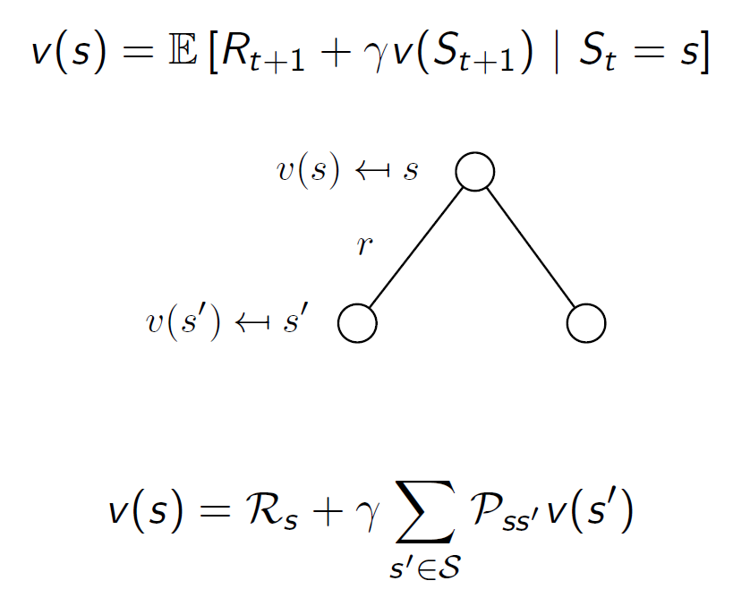• Bellman Equation for Student MRP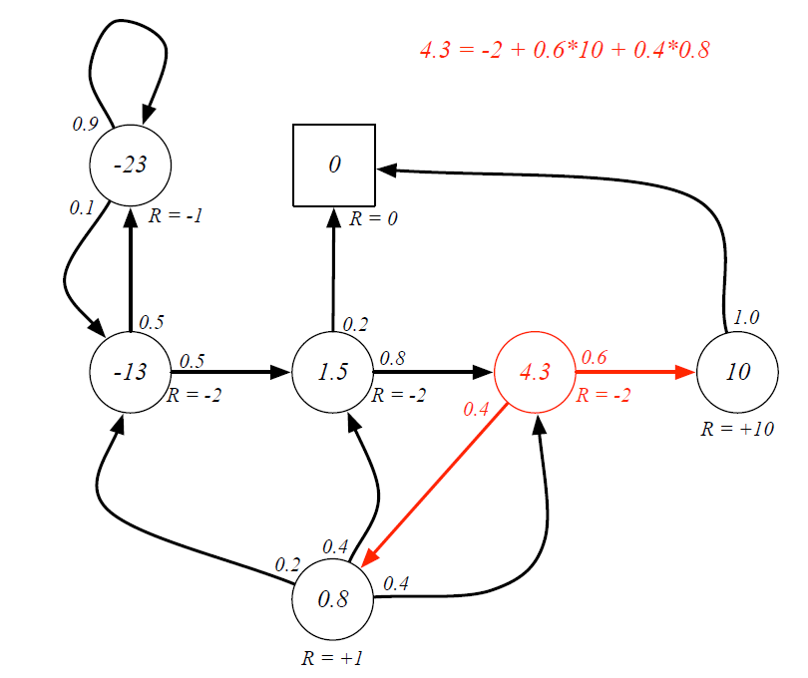## 2.1. Bellman Equation in Matrix Form¶

$$v(s) = R + \gamma \sum_{s' \in S} P_{ss'}v\left(s'\right) \qquad \forall s$$

The Bellman equation can be expressed concisely using matrices,

$$v = R + \gamma P v$$

where $v$ is a column vector with one entry per state

$$\begin{bmatrix} v(1)\\ \vdots \\v(n) \end{bmatrix} = \begin{bmatrix} R_1\\ \vdots \\R_n \end{bmatrix} + \gamma \begin{bmatrix} p_{11} & \cdots & p_{1n}\\ &\vdots& \\p_{n1}& \cdots &p_{nn} \end{bmatrix} \begin{bmatrix} v(1)\\ \vdots \\v(n) \end{bmatrix}$$

## 2.2. Solving the Bellman Equation¶

• The Bellman equation is a linear equation
• It can be solved directly:

\begin{align*} v &= R + \gamma P v \\ (I-\gamma R) v & = R \\ v & = (I - \gamma P)^{-1}R \end{align*}

• Direct solution only possible for small MRP
• Computational complexity is $O(n^3)$ for $n$ states• There are many iterative methods for large MRP
• Dynamic programming
• Monte-Carlo simulation
• Temporal-Difference learning
• Iterative algorithm for value function (Value Iteration)

• Initialize $v_1(s) = 0$ for all $s$

• For $k=1$ until convergence

• for all $s$ in $S$
$$v_{k+1}(s) \;\; \longleftarrow \;\; R(s) + \gamma \sum_{s' \in S} p\left(s' \mid s\right) v_k \left(s'\right)$$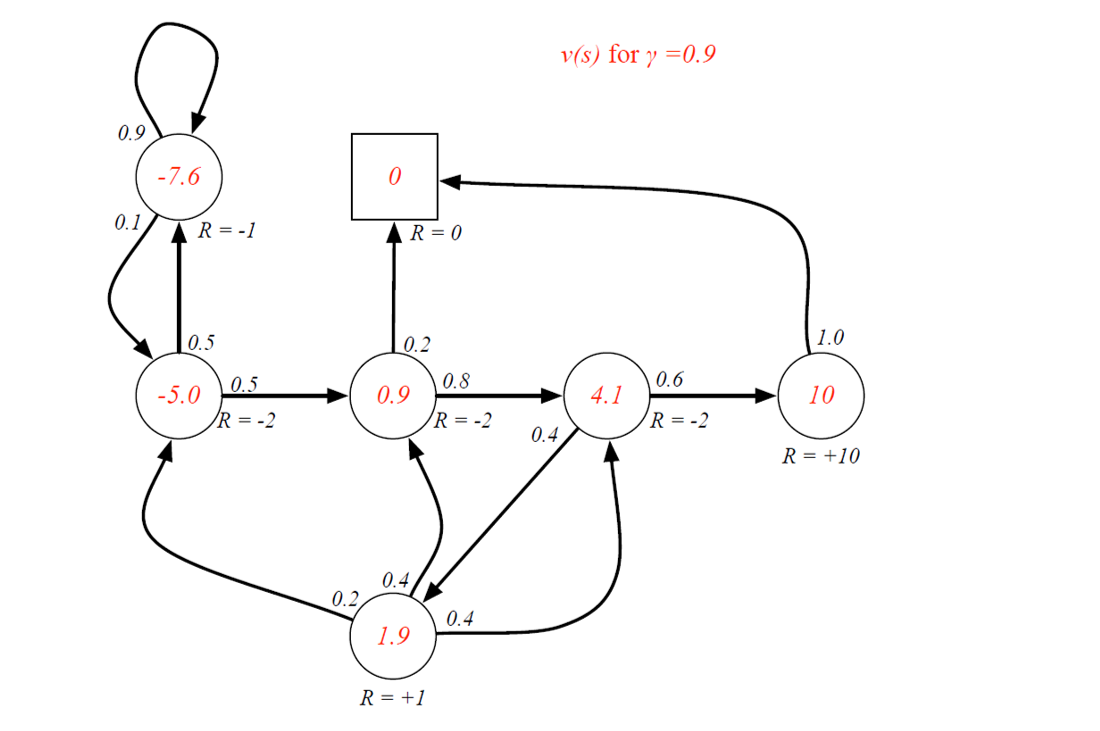In :
# [C1 C2 C3 Pass Pub FB Sleep] = [0 1 2 3 4 5 6]

P = [[0, 0.5, 0, 0, 0, 0.5, 0],
[0, 0, 0.8, 0, 0, 0, 0.2],
[0, 0, 0, 0.6, 0.4, 0, 0],
[0, 0, 0, 0, 0, 0, 1],
[0.2, 0.4, 0.4, 0, 0, 0, 0],
[0.1, 0, 0, 0, 0, 0.9, 0],
[0, 0, 0, 0, 0, 0, 1]]

R = [-2, -2, -2, 10, 1, -1, 0]

P = np.asmatrix(P)
R = np.asmatrix(R)
R = R.T

gamma = 0.9

v = (np.eye(7) - gamma*P).I*R
print(v)

[[-5.01272891]
[ 0.9426553 ]
[ 4.08702125]
[10.        ]
[ 1.90839235]
[-7.63760843]
[ 0.        ]]

In :
gamma = 0.9

v = np.zeros([7,1])

for i in range(50):
v = R + gamma*P*v

print(v)

[[-5.01073295]
[ 0.9428713 ]
[ 4.08727922]
[10.        ]
[ 1.90900857]
[-7.63401027]
[ 0.        ]]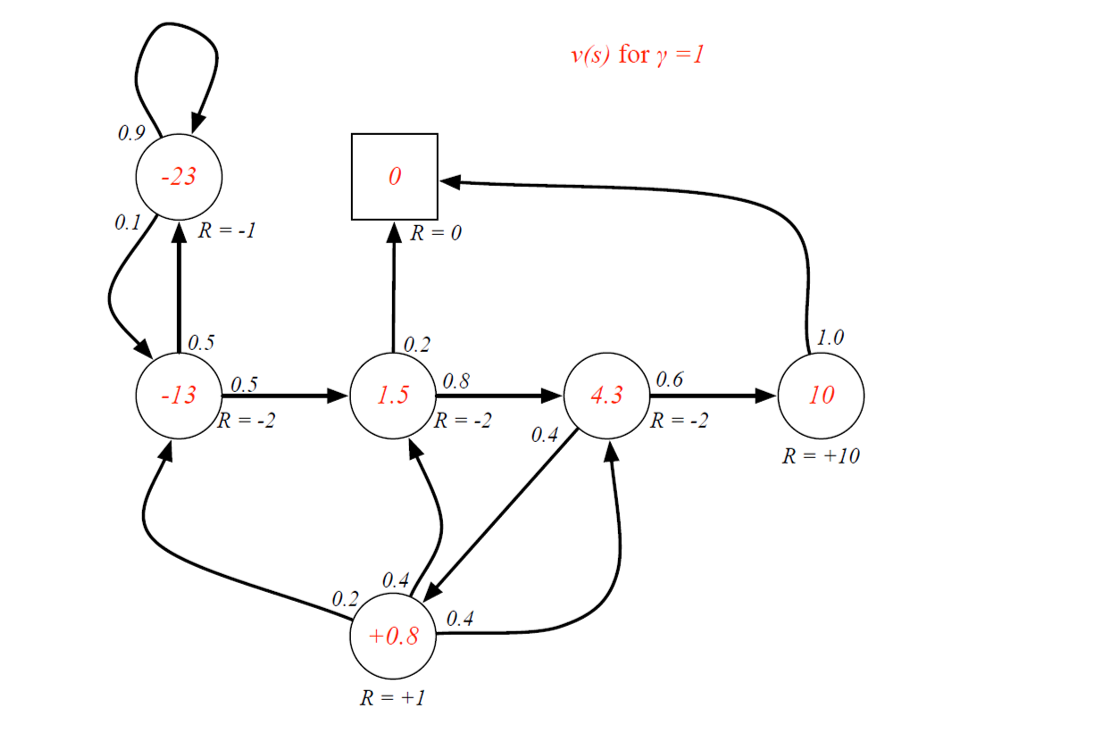In :
gamma = 1

v = np.zeros([7,1])

for i in range(50):
v = R + gamma*P*v

print(v)

[[-11.32491882]
[  1.58863104]
[  4.47845046]
[ 10.        ]
[  1.17859723]
[-20.34696584]
[  0.        ]]

In [ ]:
%%javascript
\$.getScript('https://kmahelona.github.io/ipython_notebook_goodies/ipython_notebook_toc.js')